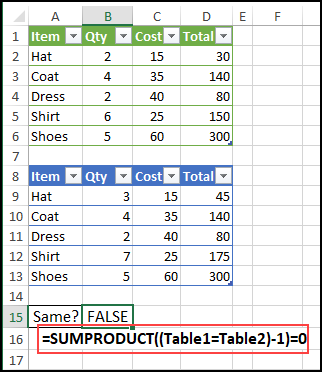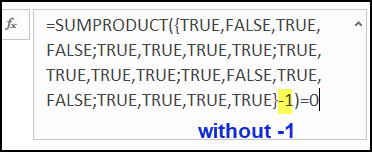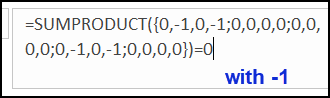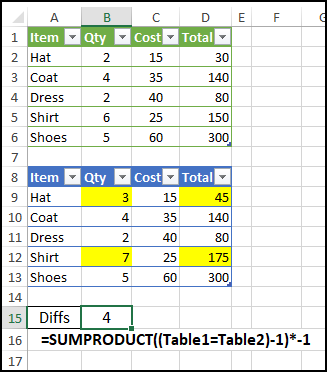# Compare Two Excel Tables with Simple FormulaDo you ever need to compare two Excel Tables? Here's a simple formula that quickly shows if there are any differences, between tables that have the same number of columns and rows.

### Compare Two Tables

This simple, and very short, formula will compare two Excel Tables, that should be identical, but might have differences. Maybe one or two numbers have changed, but those little differences aren't easy to see.

With this formula, you won't have to manually comparing the tables, row by row. And you won't need to create columns full of formulas to look for differences.

Thanks to UniMord for this tip!

### Simple Formula

Just enter this formula, somewhere on the worksheet, using the names of your tables, where I have Table1 and Table2.

=SUMPRODUCT((Table1=Table2)-1)=0

The formula result is TRUE, if the tables are exactly the same. If there are any differences, the result is FALSE.### SUMPRODUCT Comparisons

First, the SUMPRODUCT function multiplies the array of table value comparisons, and returns the sum.

SUMPRODUCT((Table1=Table2)-1)

Next, the formula tests to see if the sum is equal to zero.

=0

### Why Subtract 1?

Do you know why there is "-1" in the formula?

This formula compares each cell in the two tables. and we can see the array of results in the Formula Bar.

If you highlight just this part of the formula -- (Table1=Table2) -- in the Formula Bar, and press F9 to calculate, you'll see all the results as TRUE or FALSE### Convert to Numbers

However, if you also include the -1, and the press F9 again, that arithmetic operation changes the results to numbers.If all the comparisons are TRUE (1),

• all the numbers will be zero (1-1)
• the total will be zero

If any comparisons are FALSE (0)

• those differences will show as -1 (0-1)
• the total will not be zero.

### How Many Differences?

To see how many differences there are between the two tables, you can make a slight change to the formula.

Instead of testing if the sum is equal to zero, multiply the sum by -1.

=SUMPRODUCT((Table1=Table2)-1)*-1

In this example, there are four differences between the two tables.### More SUMPRODUCT Examples

There's lots that you can do with the under-appreciated SUMPRODUCT function. See more SUMPRODUCT examples on my Contextures website.

_________________

### Compare Two Excel Tables with Simple Formula_________________

## 7 thoughts on “Compare Two Excel Tables with Simple Formula”

1. Thanks, Jan Karel, and have you written an article about it yet, with more details?

1. Jeremy says:

Hi, Debra. Love your site and your newsletters. I'm confused by this one, though, and wanted to make sure I wasn't missing anything. This formula works for very small tables but isn't at all practical for real-world use. When I'm comparing tables at work, I'm looking at thousands of rows of data...not 5. And knowing if there are differences and how many differences there are doesn't really help. I need to know which specific cells are incorrect, so I can correct them somehow. I haven't really found an elegant solution yet...mostly because this type of exercise happens to infrequently, it hasn't been worth the time to think about it.

1. Thanks, Jeremy, and you're right - this formula won't help in large tables where you know there are differences. It's a quick check for tables that should be the same.
Perhaps you need to make sure that the list in workbook A is the latest version, so you compare it to the master list in workbook B.
You could try Jan Karel's new table comparison tool - there's a link in his comment, above.

2. Shairal says:

This is a quick way to find out if something's different - great idea!
I also started using Inquire (I have Excel 365) and it does a great job at comparing and highlighting differences.

Thanks for the formula and the explanation! 🙂

3. I've got a utility for comparing workbooks as part of my personal macro workbook (shared with my co-workers) that has a little form for the front end and runs on an AND formula like:
=AND(Table1=Table2).
We use it on a daily basis for validating before-and-after results of edited queries. If they don't match I either start entering similar formulas on a column-by-column basis, or using my utility that pivots the two tables together *. For some reason I never use the built-in Excel tools, although I've told other people about them.
Your SUMPRODUCT is nice since it doesn't have to be entered as an array formula.

This site uses Akismet to reduce spam. Learn how your comment data is processed.